# Area of a circle inscribed in a rectangle which is inscribed in a semicircle?

Let us consider one semicircle is given. Its radius is R. One rectangle of length l and breadth b is inscribed in that semi-circle. Now one circle with radius r is inscribed in the rectangle. We have to find the area of the inner circle.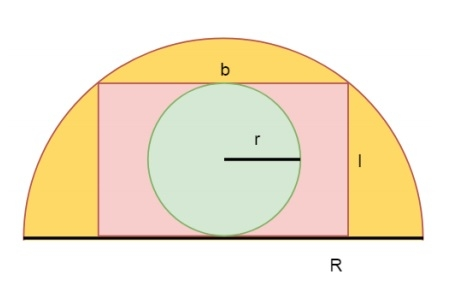As we know biggest rectangle that can be inscribed within the semi-circle has length l and breadth b, then the equation of l and b will be like following −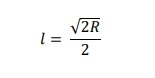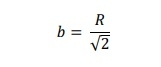Now, the biggest circle that can be inscribed within the rectangle has radius r is like below −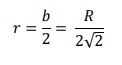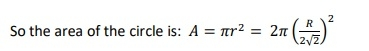## Example

#include <iostream>
#include <cmath>
using namespace std;
float innerCircleArea(float R){
return 3.1415 * pow(R / (2 * sqrt(2)), 2);
}
int main() {
}
Area: 56.547